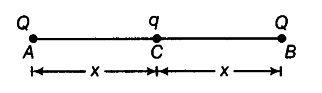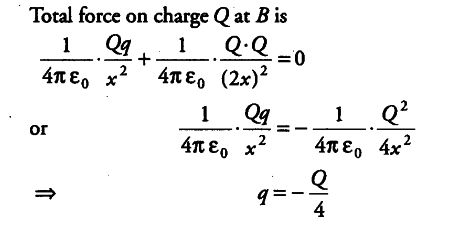# A charge q is placed at the centre of the line joining two equal charges

A charge q is placed at the centre of the line joining two equal charges (Q). Show that the system of three charges will be in equilibrium,
q = - Q/4

Suppose the three charges be placed as shown in the figure.As, the net force on q is zero, so it is already in equilibrium. For equilibrium of other two charges-, the net force on each charge must be zero.